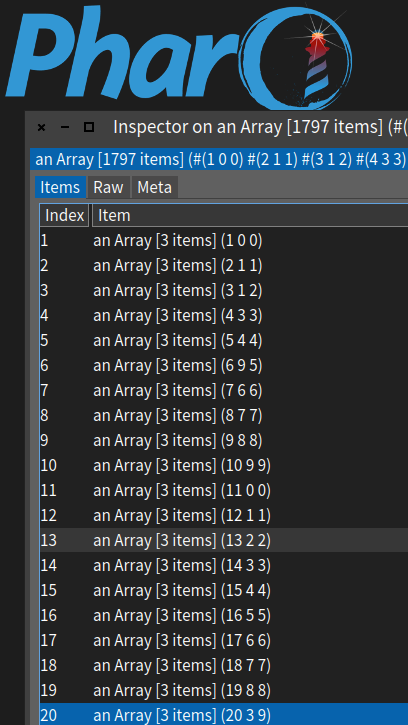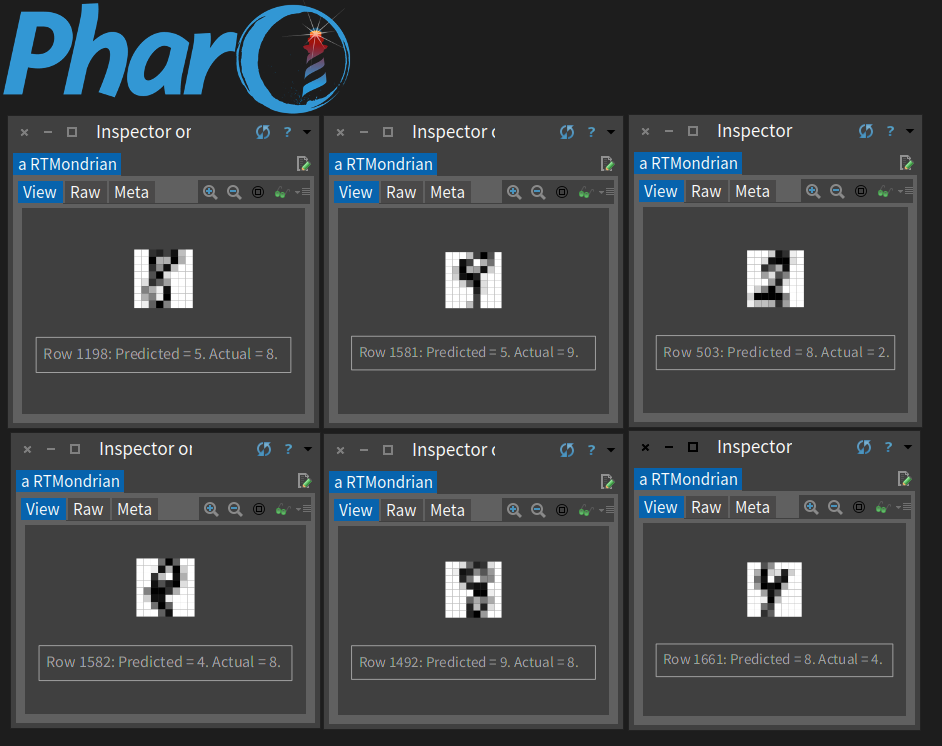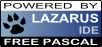## Hello, digits!

04 Feb 2018

The Hello World intro to machine learning is usually by way of the Iris flower image classification or the MNIST handwritten digit recognition. In this post, I describe training a neural network in Pharo to perform handwritten digit recognition. Instead of the MNIST dataset, I'll use the smaller UCI dataset. According to the description, this dataset consists of two files:

• optdigits.tra, 3823 records
• optdigits.tes, 1797 records
• Each record consists of 64 inputs and 1 class attributes.
• Input attributes are integers in the range 0..16.
• The class attribute is the class code 0..9, i.e., it denotes the digit that the 64 input attributes encode.

The files are in CSV format. Let's use the excellent NeoCSV package to read the data:

``````| rb training testing |

rb := [ :fn |
| r |
1 to: 64 do: [ :i |
r addFieldConverter: [ :string | string asInteger / 16.0 ]].
"The converter normalizes the 64 input integers into floats between 0 and 1."
r upToEnd ].

training := rb value: '/tmp/optdigits.tra'.
testing := rb value: '/tmp/optdigits.tes'.
``````

Next, install MLNeuralNetwork by Oleksandr Zaytsev:

``````Metacello new
repository: 'http://smalltalkhub.com/mc/Oleks/NeuralNetwork/main';
configuration: 'MLNeuralNetwork';
version: #development;
``````

MLNeuralNetwork operates on MLDataset instances, so modify the CSV reader accordingly:

``````| ohv rb dsprep training testing |

"Create and cache the 10 one-hot vectors."
ohv := IdentityDictionary new.
0 to: 9 do: [ :i |
ohv at: i put: (MLMnistReader onehot: i) ].

"No change."
rb := [ :fn |
| r |
1 to: 64 do: [ :i |
r addFieldConverter: [ :string | string asInteger / 16.0 ]].
"The converter normalizes the 64 input integers into floats between 0 and 1."
r upToEnd ].

"Turn the output of 'rb' into a MLDataset."
dsprep := [ :aa |
MLDataset new
input: (aa collect: [ :ea | ea allButLast asPMVector ])
output: (aa collect: [ :ea | ohv at: ea last ]) ].

training := dsprep value: (rb value: '/tmp/optdigits.tra').
testing := dsprep value: (rb value: '/tmp/optdigits.tes').
``````

Note MLMnistReader>>onehot: which creates a 'one-hot' vector for each digit. One-hot vectors make machine learning more effective. They are easy to understand "pictorially":

• For the digit 0, [1 0 0 0 0 0 0 0 0 0].
• For the digit 1, [0 1 0 0 0 0 0 0 0 0].
• For the digit 3, [0 0 0 1 0 0 0 0 0 0].
• ...
• For the digit 9, [0 0 0 0 0 0 0 0 0 1].

Since there are over 5,000 records, we precompute the one-hot vectors and reuse them, instead of creating one vector per record.

Now create a 3-layer neural network of 64 input, 96 hidden, and 10 output neurons, set it to learn from the training data for 500 epochs, and test it:

``````| net result |
net := MLNeuralNetwork new initialize: #(64 96 10).
net costFunction: (MLSoftmaxCrossEntropy  new).
net outputLayer activationFunction: (MLSoftmax new).
net learningRate: 0.5.
net learn: training epochs: 500.

result := Array new: testing size.
testing input doWithIndex: [ :ea :i |
| ret |
ret := net value: ea.
result at: i
put: (Array
"'i' identifies the record in the test set."
with: i
"The neuron that is 'most activated' serves as the prediction."
with: (ret indexOf: (ret max: [ :x | x ])) - 1
"The actual digit from the test set, decoded from its one-hot vector."
with: ((testing output at: i) findFirst: [ :x | x = 1 ]) - 1) ].
result inspect.
``````

From the inspector, we can see that the network got records 3, 6 and 20 wrong:In the inspector's code pane, the following snippet shows that the network's accuracy is about 92%.

``````1 - (self select: [ :x | (x second = x third) not ]) size / 1797.0)
``````

Not bad for a first attempt. However, the data set's source states that the K-Nearest Neighbours algorithm achieved up to 98% accuracy on the testing set, so there's plenty of room for improvement for the network.

Here's a screenshot showing some of the 8x8 digits with their predicted and actual values. I don't know about you, but the top right "digit" looks more like a smudge to me than any number.Code to generate the digit images follows:

``````| mb |
mb := [ :array :row :predicted :actual |
| b lb |
b := RTMondrian new.
b shape box
size: 1;
color: Color gray.
b nodes: array.
b normalizer normalizeColorAsGray: [ :x | x * 16 ].
b layout gridWithPerRow: 8;
gapSize: 0.
b build.
lb := RTLegendBuilder new.
lb view: b view.
lb textSize: 9;
': Predicted = ', predicted asString,
'. Actual = ', actual asString,
'.'.
lb build.
b ].

rand := Random new.
6 timesRepeat: [
| x |
x := rand nextInt: 1797.
(mb value: (testing input at: x)
value: x
value: (result at: x) second
value: (result at: x) third)
inspect ]
``````

Let's also look at the failed predictions:

``````| failed |
failed := (result select: [ :x | (x second = x third) not ])
collect: [ :x | x first ].
6 timesRepeat: [
| x |
x := rand nextInt: 1797.
[ failed includes: x ] whileFalse: [ x := rand nextInt: 1797 ].
(mb value: (testing input at: x)
value: x
value: (result at: x) second
value: (result at: x) third)
inspect ]
``````Putting the code altogether:

``````| ohv rb dsprep training testing net result mb rand failed |

ohv := IdentityDictionary new.
0 to: 9 do: [ :i |
ohv at: i put: (MLMnistReader onehot: i) ].

rb := [ :fn |
| r |
1 to: 64 do: [ :i |
r addFieldConverter: [ :string | string asInteger / 16.0 ]].
r upToEnd ].

dsprep := [ :aa |
MLDataset new
input: (aa collect: [ :ea | ea allButLast asPMVector ])
output: (aa collect: [ :ea | ohv at: ea last ]) ].

training := dsprep value: (rb value: '/tmp/optdigits.tra').
testing := dsprep value: (rb value: '/tmp/optdigits.tes').

net := MLNeuralNetwork new initialize: #(64 96 10).
net costFunction: (MLSoftmaxCrossEntropy  new).
net outputLayer activationFunction: (MLSoftmax new).
net learningRate: 0.5.
net learn: training epochs: 500.

result := Array new: testing size.
testing input doWithIndex: [ :ea :i |
| ret |
ret := net value: ea.
result at: i
put: (Array
"'i' identifies the record in the test set."
with: i
"The neuron that is 'most activated' serves as the prediction."
with: (ret indexOf: (ret max: [ :x | x ])) - 1
"The actual digit from the test set, decoded from its one-hot vector."
with: ((testing output at: i) findFirst: [ :x | x = 1 ]) - 1) ].
result inspect.

mb := [ :array :row :predicted :actual |
| b lb |
b := RTMondrian new.
b shape box
size: 1;
color: Color gray.
b nodes: array.
b normalizer normalizeColorAsGray: [ :x | x * 16 ].
b layout gridWithPerRow: 8;
gapSize: 0.
b build.
lb := RTLegendBuilder new.
lb view: b view.
lb textSize: 8;
': Predicted = ', predicted asString,
'. Actual = ', actual asString,
'.'.
lb build.
b ].

rand := Random new.
6 timesRepeat: [
| x |
x := rand nextInt: 1797.
(mb value: (testing input at: x)
value: x
value: (result at: x) second
value: (result at: x) third)
inspect ].

failed := (result select: [ :x | (x second = x third) not ]) collect: [ :x | x first ].
6 timesRepeat: [
| x |
x := rand nextInt: 1797.
[ failed includes: x ] whileFalse: [ x := rand nextInt: 1797 ].
(mb value: (testing input at: x)
value: x
value: (result at: x) second
value: (result at: x) third)
inspect ]
``````
Tags: deep learning, machine learning